Letters for Digits - Addition Questions

Chapter 16 Class 8 Playing with Numbers
Concept wise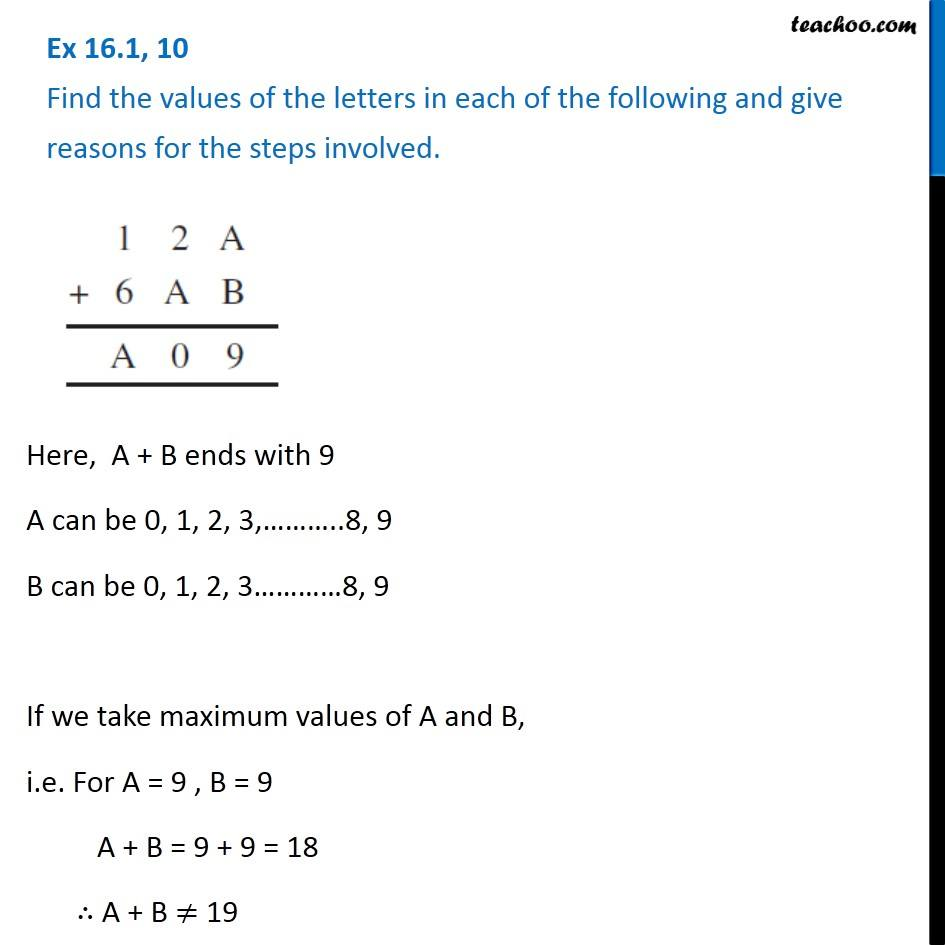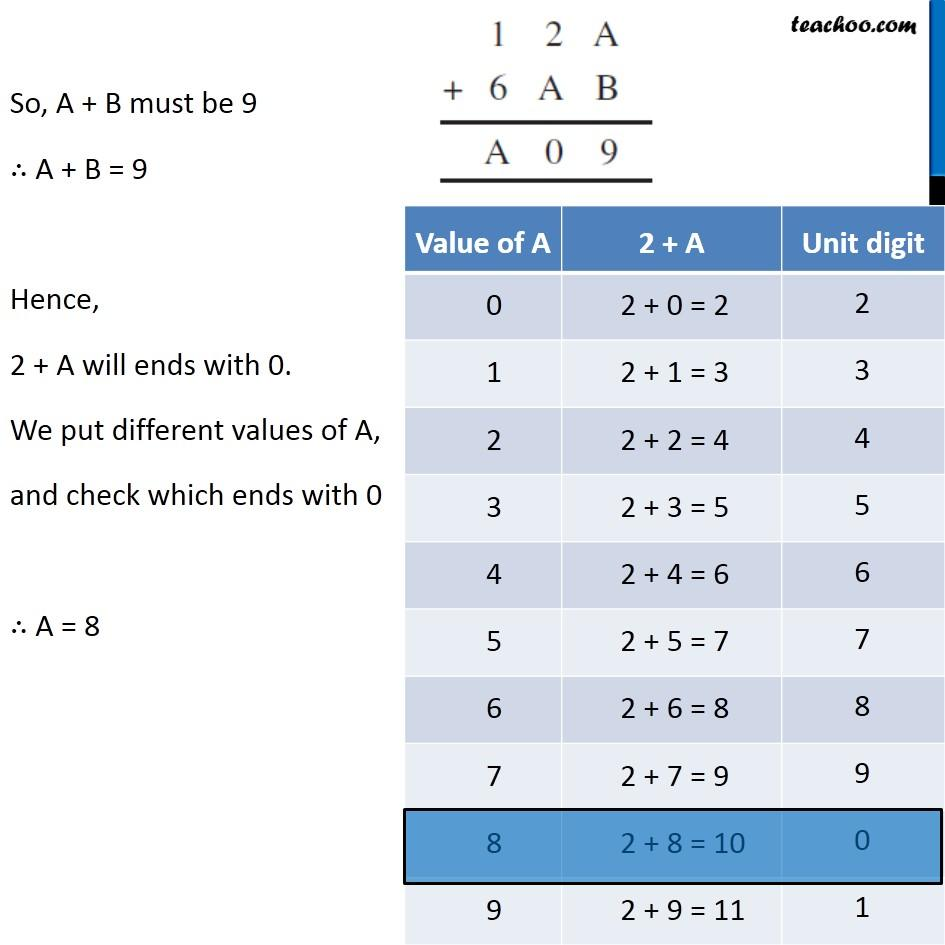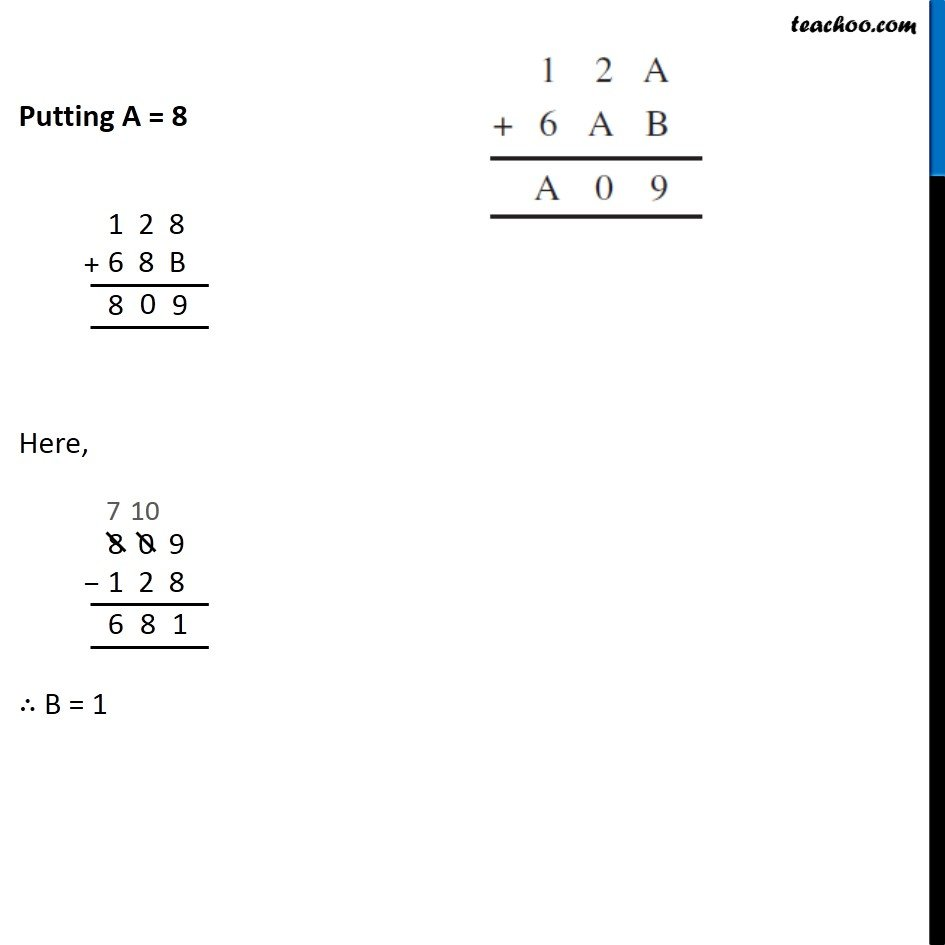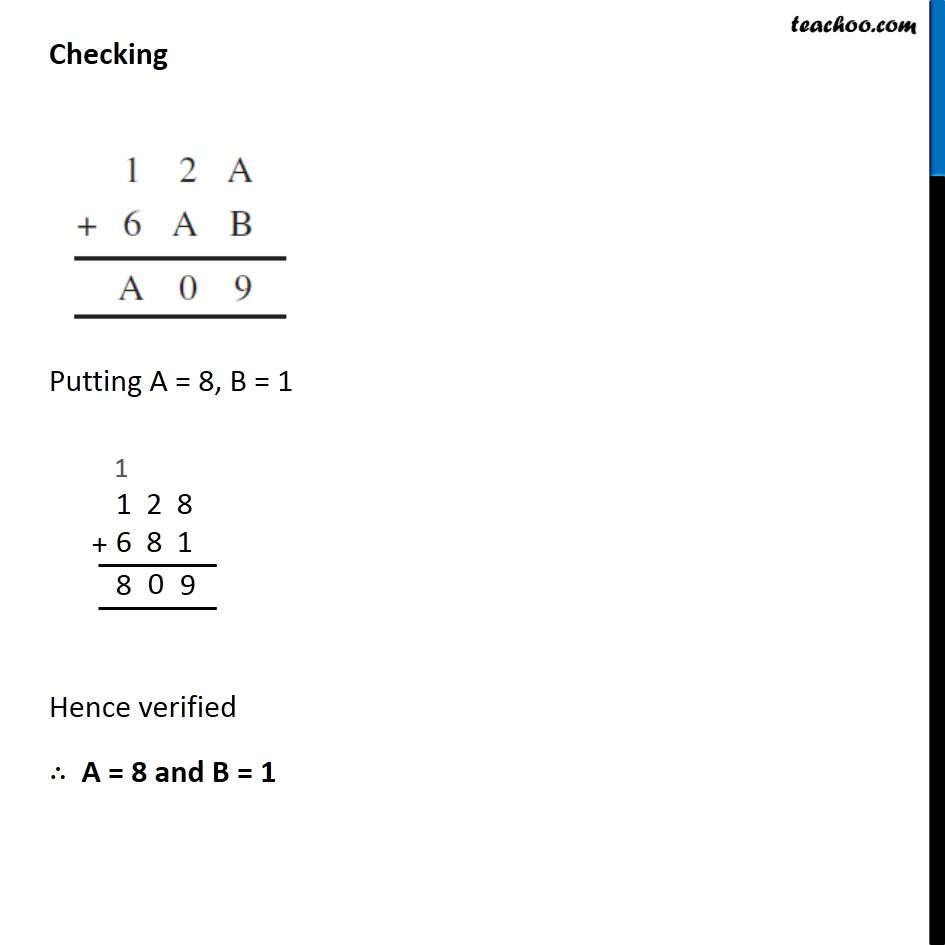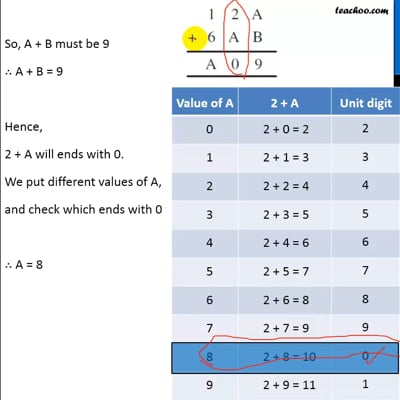This video is only available for Teachoo black users

Introducing your new favourite teacher - Teachoo Black, at only ₹83 per month

### Transcript

Ex 16.1, 10 Find the values of the letters in each of the following and give reasons for the steps involved. Here, A + B ends with 9 A can be 0, 1, 2, 3,………..8, 9 B can be 0, 1, 2, 3…………8, 9 If we take maximum values of A and B, i.e. For A = 9 , B = 9 A + B = 9 + 9 = 18 ∴ A + B ≠ 19 So, A + B must be 9 ∴ A + B = 9 Hence, 2 + A will ends with 0. We put different values of A, and check which ends with 0 ∴ A = 8 Putting A = 8 Here, ∴ B = 1 Checking Putting A = 8, B = 1 Hence verified ∴ A = 8 and B = 1